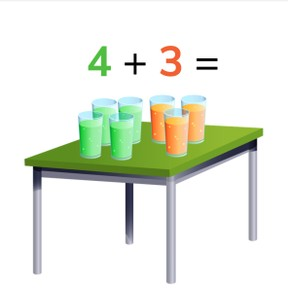No account needed.8,000 schools use Gynzy92,000 teachers use Gynzy1,600,000 students use Gynzy

-K.OA.A.1
-K.OA.A.2

## Relevance

It is important that students are able to add to 10 so they can tell how many they have of an object. An example is if there are 3 and 4 glasses of soda, they know that there are 7 together.

## Introduction

Practice counting amounts with students and discuss how to count amounts. Then practice their knowledge of numbers by asking which numbers are shown on the interactive whiteboard.

## Development

With some addition problems the amounts are visible as numbers of objects that you can count. Explain to students that they can solve these addition problems by first counting the objects in the first group, and then to count on with the objects in the second group to reach their total. Say that the first number is always the amount you already have, and the second number is the amount you are adding. Practice with a few examples.
If the numbers of an addition problem are not visible as countable objects, you can use blocks or a rekenrek to solve the problem. They can also use the number line. Discuss that you can calculate addition problems in different ways, for example by swapping the numbers in the problem, or by solving a problem that is one more or one less. Practice these different ways of calculation with the students with a few addition problems, and make sure to ask students how they are solving the problems.
Next discuss with students how to solve a story problem. First students must look at what is happening, then to determine which numbers are required for the story problem, to write out the math problem, and then to solve the addition problem. Practice this with a few examples.

Check that students can add to 10 by asking the following questions:
- What does adding do? (count things together)
- What do you do to add? (count first number and second number together for a larger total)
- Can you swap the numbers in an addition problem?

## Guided practice

The first problems ask students to solve problems in which the numbers are visually represented by objects. Then students get an exercise in which they must fill in the correct answer. The final exercise asks students to solve a story problem, where students are asked to determine the addition problem based on the story.

## Closing

Discuss with students that it is important to be able to add to 10 so they can calculate how many they have together of an obuject. PRactice different addition problems to 10.

## Teaching tips

Students who have difficulty with abstract addition problems can be supported by using blocks or a rekenrek.

## Instruction materials

blocks, rekenrek

### The online teaching platform for interactive whiteboards and displays in schools

• Save time building lessons

• Manage the classroom more efficiently

• Increase student engagement